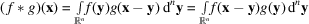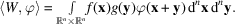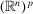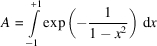International
Tables for
Crystallography
Volume B
Reciprocal space
Edited by U. Shmueli

International Tables for Crystallography (2010). Vol. B, ch. 1.3, pp. 34-35   | 1 | 2 |

## Section 1.3.2.3.9.7. Convolution of distributions

G. Bricognea

aGlobal Phasing Ltd, Sheraton House, Suites 14–16, Castle Park, Cambridge CB3 0AX, England, and LURE, Bâtiment 209D, Université Paris-Sud, 91405 Orsay, France

#### 1.3.2.3.9.7. Convolution of distributions

| top | pdf |

The convolutionof two functions f and g onis defined bywhenever the integral exists. This is the case when f and g are both in; thenis also in. Let S, T and W denote the distributions associated to f, g andrespectively: a change of variable immediately shows that for any,Introducing the map σ fromtodefined by, the latter expression may be written:(wheredenotes the composition of mappings) or by a slight abuse of notation:A difficulty arises in extending this definition to general distributions S and T because the mapping σ is not proper: if K is compact in, thenis a cylinder with base K and generator the second bisector'in. However,is defined whenever the intersection between Suppandis compact.

We may therefore define the convolutionof two distributions S and T onbywhenever the following support condition is fulfilled:

the setis compact infor all K compact in'.

The latter condition is met, in particular, if S or T has compact support. The support ofis easily seen to be contained in the closure of the vector sumConvolution by a fixed distribution S is a continuous operation for the topology on: it maps convergent sequencesto convergent sequences. Convolution is commutative:.

The convolution of p distributionswith supportscan be defined bywhenever the following generalized support condition:

the setis compact infor all K compact in'

is satisfied. It is then associative. Interesting examples of associativity failure, which can be traced back to violations of the support condition, may be found in Bracewell (1986, pp. 436–437).

It follows from previous definitions that, for all distributions, the following identities hold:

 (i):is the unit convolution; (ii): translation is a convolution with the corresponding translate of δ; (iii): differentiation is a convolution with the corresponding derivative of δ; (iv) translates or derivatives of a convolution may be obtained by translating or differentiating any one of the factors: convolution commutes' with translation and differentiation, a property used in Section 1.3.4.4.7.7to speed up least-squares model refinement for macromolecules.

The latter property is frequently used for the purpose of regularization: if T is a distribution, α an infinitely differentiable function, and at least one of the two has compact support, thenis an infinitely differentiable ordinary function. Since sequencesof such functions α can be constructed which have compact support and converge to δ, it follows that any distribution T can be obtained as the limit of infinitely differentiable functions. In topological jargon:is `everywhere dense' in. A standard function inwhich is often used for such proofs is defined as follows: putwith(so that θ is inand is normalized), and putAnother related result, also proved by convolution, is the structure theorem: the restriction of a distributionto a bounded open set Ω inis a derivative of finite order of a continuous function.

Properties (i)to (iv)are the basis of the symbolic or operational calculus (see Carslaw & Jaeger, 1948; Van der Pol & Bremmer, 1955; Churchill, 1958; Erdélyi, 1962; Moore, 1971) for solving integro-differential equations with constant coefficients by turning them into convolution equations, then using factorization methods for convolution algebras (Schwartz, 1965).

### References

Bracewell, R. N. (1986). The Fourier Transform and its Applications, 2nd ed., revised. New York: McGraw-Hill.
Carslaw, H. S. & Jaeger, J. C. (1948). Operational Methods in Applied Mathematics. Oxford University Press.
Churchill, R. V. (1958). Operational Mathematics, 2nd ed. New York: McGraw-Hill.
Erdélyi, A. (1962). Operational Calculus and Generalized Functions. New York: Holt, Rinehart & Winston.
Moore, D. H. (1971). Heaviside Operational Calculus. An Elementary Foundation. New York: American Elsevier.
Schwartz, L. (1965). Mathematics for the Physical Sciences. Paris: Hermann, and Reading: Addison-Wesley.
Van der Pol, B. & Bremmer, H. (1955). Operational Calculus, 2nd ed. Cambridge University Press.